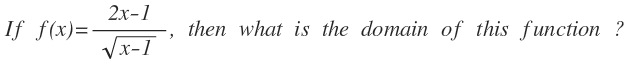# Domain of a functionIf $f(x) = \dfrac{2x-1}{\sqrt{x-1}}$, then what is the domain of this function?

×